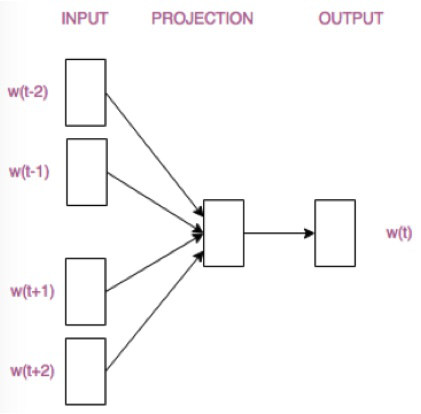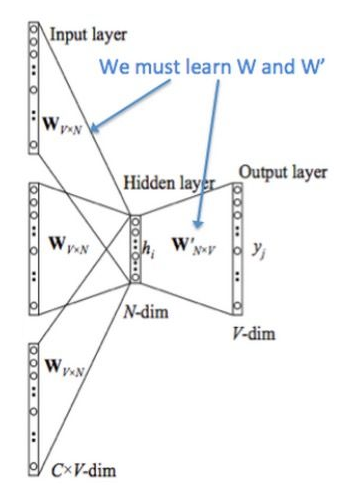# python 自带的word2vec讲解_word2vec训练

python 自带的word2vec讲解_word2vec训练word2vec理解学习nlp最先了解的概念应该就是词嵌入（wordembedding）吧，Word2vec是谷歌于2013年提出的一种有效的词嵌入的方法，采用了两种模型(CBOW与skip-gram模型)与两种优化方法(负采样与层次softmax方法)的组合。现在使用Word2vec获得词的向量表达，并将其应用于各种nlp任务中已经非常常见。由于我们要用计算机来完成各种自然语言理解的任务，而…

## word2vec理解

##### One-hot Representation

“易烊千玺”表示为：[1 0 0 0]
“喜欢”表示为：[0 1 0 0]
“我”表示为：[0 0 1 0]
“李现”表示为:[0 0 0 1]

One-hot Representation这种将一个词转化成一个高维稀疏向量的表示方式比较简单，但缺点也很明显。一个缺陷就是容易造成维度灾难，如果语料中有五万个词，那么每个词向量就是五万维，然而平时用来训练的语料词语数都很多，数量成千上万很常见。另一个缺陷是这种方法无法表示词之间的相关性，每个词之间都是独立的，不包含语义信息无法反映出词语在语义层面的含义。

##### distributed representation

Word2vec这种词嵌入的方法在训练的过程中考虑了词语的上下文，就是在目标词（x）和目标词的上下文(y)之间建立一个语言模型f(x)=y，对这个语言模型进行训练，从而实现根据上下文的词得到目标词或根据目标词得到其上下文的词。可以说一个词由其上下文的词表示，而相似的词往往拥有相似的上下文语境，所以相似的词用这种方式得到的词向量也相似。
Word2vec用神经网络的方式来建立上下文和目标词之间的语言模型，当神经网络训练结束后，其隐藏层的权重就是我们要的每个词的词向量。所以word2vec是一个通过训练神经网络得到语言模型的任务，只不过这个任务是个幌子，我们实际要的是这个过程中产生的副产物即隐藏层权重，用这个副产物作为每个词的词向量，将获得的词向量送入下游nlp任务中进行应用才是最终目的。

## CBOW

#### CBOW模型理解

CBOW模型根据某个中心词前后A个连续的词，来计算该中心词出现的概率，即用上下文预测目标词。模型结构简易示意图如下：1.在输入层，输入的是多个上下文单词的one-hot。
(维度：因为corpus中有V个单词，所以每个单词的one-hot的维度1*V，那么在输入层输入的就是C个1*V的向量，所以在输入层，数据维度为C*V)
2.设定最终获得的词向量的维度为N，初始化输入层与隐藏层之间的权重矩阵w，w维度为V*N。上下文单词的one-hot(C*V)与网络的输入权重矩阵w(V*N)相乘，得到C个1*N的向量。把它们求和再平均，得到隐藏层向量h，维度为1*N.
h = 1 C ( x 1 + x 2 + . . . + x c ) ⋅ w h = \frac{1}{C}({x_1} + {x_2} + … + {x_c}) \cdot w
3.初始化隐藏层与输出层之间的权重矩阵 w , {w^,} ,维度为N*V。
4.隐藏层向量h(1*N)与 w ′ {w\prime} (N*V)相乘，得到1*V的向量u， u = h ⋅ w ′ u = h \cdot w\prime 。为了方便概率表示,将向量u经过softmax，此时向量softmax(u)的每一维代表语料中的一个单词。向量softmax(u)概率最大的位置所代表的单词为模型预测出的中间词。
5.上一步输出的1*V向量与groud truth中的one hot比较。训练的目的是最大化实际中心词出现的概率，基于此定义损失函数，通过最小化损失函数，采用梯度下降算法更新W和W’。当网络收敛则训练完成，此时矩阵W就是我们想要的词向量。如果我们想要知道语料中某个词的向量表示，就用这个词的one-hot乘以权重矩阵w，得到1*N的向量，这个向量就是我们要找的这个词的向量表示。

#### CBOW模型参数训练过程

##### 前向传播：

Input-hidden权重矩阵： w

h = 1 C w ⊤ ( x 1 + x 2 + . . . + x c ) = 1 C ( v W , I 1 + v W , I 2 + . . . + v W , I c ) ⊤ h = \frac{1}{C}{w^ \top }({x_1} + {x_2} + … + {x_c}) = \frac{1}{C}{({v_{W,I1}} + {v_{W,I2}} + … + {v_{W,Ic}})^ \top }
Hidden-output权重矩阵： w’

p ( w j ∣ w I 1 , w I 2 , . . . w I C ) = y j = exp ⁡ ( u j ) ∑ j ′ = 1 v exp ⁡ ( u j ′ ) p({w_j}|{w_{I1,}}{w_{I2,}}…{w_{IC}}) = {y_j} = \frac{
{\exp ({u_j})}}{
{\sum\limits_{j\prime = 1}^{\text{v}} {\exp ({u_{j\prime }})} }}

max ⁡ p ( w O ∣ w I 1 , w I 2 , . . . w I C ) = max ⁡ log ⁡ p ( w O ∣ w I 1 , w I 2 , . . . w I C ) = max ⁡ log ⁡ exp ⁡ ( u j ∗ ) ∑ j ′ = 1 v exp ⁡ ( u j ′ ) = max ⁡ ( u j ∗ − log ⁡ ∑ j ′ = 1 v exp ⁡ ( u j ′ ) ) \begin{array}{l} \max p({w_O}|{w_{I1,}}{w_{I2,}}…{w_{IC}})\\ {\rm{ = }}\max \log p({w_O}|{w_{I1,}}{w_{I2,}}…{w_{IC}})\\ = \max \log \frac{
{\exp ({u_{
{j^*}}})}}{
{\sum\limits_{_{j\prime } = 1}^{\rm{v}} {\exp ({u_{j\prime }})} }} = \max ({u_{
{j^*}}} – \log \sum\limits_{_{j\prime } = 1}^{\rm{v}} {\exp ({u_{j\prime }})} ) \end{array}

E = − log ⁡ p ( w O ∣ w I 1 , w I 2 , . . . w I C ) = log ⁡ ∑ j ′ = 1 v exp ⁡ ( u j ′ ) − u j ∗ = log ⁡ ∑ j ′ = 1 v exp ⁡ ( v ′ w j ⊤ ⋅ h ) − v ′ w o ⊤ ⋅ h E = – \log p({w_O}|{w_{I1,}}{w_{I2,}}…{w_{IC}}) = \log \sum\limits_{j\prime = 1}^{\text{v}} {\exp ({u_{j\prime }})} – u{}_{
{j^*}} = \log \sum\limits_{j\prime = 1}^{\text{v}} {\exp (v{\prime _{wj}}^ \top \cdot {\text{h}})} – v{\prime _{wo}}^ \top \cdot {\text{h}}

##### 反向传播、随机梯度下降更新权重：

1.损失函数E 对w’取导数，获得隐层到输出层的权重的梯度

∂ E ∂ w ′ = ∂ E ∂ u j ⋅ ∂ u j ∂ w ′ = e j ⋅ h \frac{
{\partial E}}{
{\partial w\prime }} = \frac{
{\partial E}}{
{\partial {u_j}}} \cdot \frac{
{\partial {u_j}}}{
{\partial w\prime }} = {e_j} \cdot h
，其中 e j {e_j} 是预测误差， e j = y j − t j {e_j} = {y_j} – {t_j}

w ′ ( n e w ) = w ′ ( o l d ) − η ⋅ e j ⋅ h , f o r   j = 1 , 2 , . . . v w{\prime ^{(new)}} = w{\prime ^{(old)}} – \eta \cdot {e_j} \cdot h,for{\text{ }}j = 1,2,…v 该方程向量方式的写法为： v w j ′ ( n e w ) = v w j ′ ( o l d ) − η ⋅ e j ⋅ h , f o r   j = 1 , 2 , . . . v {v_{wj}}{\prime ^{(new)}} = {v_{wj}}{\prime ^{(old)}} – \eta \cdot {e_j} \cdot h,for{\text{ }}j = 1,2,…v 其中 v ′ w j v{\prime _w}_j 是矩阵w’的第j列向量，η为学习率。
2.损失函数E 对w取导数，获得输入层到隐层的权重的梯度

∂ E ∂ w = ∂ E ∂ h ⋅ ∂ h ∂ w \frac{
{\partial E}}{
{\partial w}} = \frac{
{\partial E}}{
{\partial h}} \cdot \frac{
{\partial h}}{
{\partial w}}
，其中 ∂ E ∂ h = ∑ j = 1 v ∂ E ∂ u j ∂ u j ∂ h = ∑ j = 1 v e j w ′ = E H \frac{
{\partial E}}{
{\partial h}} = \sum\limits_{j = 1}^v {\frac{
{\partial E}}{
{\partial {u_j}}}} \frac{
{\partial {u_j}}}{
{\partial h}} = \sum\limits_{j = 1}^v {
{e_j}} w\prime = EH

{
{_w}_{,I}}}^ \top

#### CBOW举例

1.在训练前，首先要将原始文本生成训练样本数据。下图展示了根据原始语料生成训练数据的过程。## Skip-Gram

#### Skip-Gram模型理解

Skip-Gram与CBOW相反，它是用中心词预测其上下文的词。1.在输入层，输入的是选定的一个中心词的one-hot，维度V*1。
2.设定最终获得的词向量的维度为N，初始化输入层与隐藏层之间的权重矩阵w，维度为V*N。中心词的one-hot(V*1)与输入权重矩阵w(V*N)相乘，得到1个N*1的向量，将其作为隐藏层向量。 h = w ⊤ ⋅ x = v w I ⊤ h = {w^ \top } \cdot x = v_{wI}^ \top
3.初始化隐藏层与输出层之间的权重矩阵 w ′ w{\prime} ,维度为N*V。
4.隐藏层向量h(N*1)与 w ′ w{\prime} (N*V)相乘C次，得到 u c , j = w ′ ⊤ ⋅ h = v ′ w j ⊤ ⋅ h {u_{c,j}} = w{\prime ^ \top } \cdot h = v{\prime _{wj}}^ \top \cdot h ，为了方便概率表示,将向量 u c , j {u_{c,j}} 经过softmax，得到C个V*1的向量，每个V*1向量的每一维代表语料中的一个单词。第C个V*1向量中概率最大的位置所代表的单词，就是由中心词预测出的第c个上下文位置的词。Skip-Gram在输出层不是输出一个多项分布，而是输出C个多项分布。
5.上一步输出的C个V*1向量与C个groud truth中的one hot比较。训练的目的是最大化实际上下文词出现的概率，基于此定义损失函数，通过最小化损失函数，采用梯度下降算法更新W和W’。当网络收敛则训练完成，此时矩阵W就是我们想要的词向量。如果我们想要知道语料中某个词的向量表示，就用这个词的one-hot乘以权重矩阵w，得到N*1的向量，这个向量就是我们要找的这个词的向量表示。

### Skip-Gram模型参数训练过程

##### 前向传播：

Hidden-output权重矩阵： w ′ w{\prime}

{\text{wj}}}}
w ′ w{\prime} 的第j列向量。由于每个输出的节点共享相同的 w ′ w{\prime} ，所以 u c j = u j {u_{cj}} = {u_j}

p ( w c , j = w o , c ∣ w I ) = y c , j = exp ⁡ ( u c , j ) ∑ j ′ = 1 v exp ⁡ ( u j ′ ) p({w_{c,j}} = {w_{o,c}}|{w_I}) = {y_{c,j}} = \frac{
{\exp ({u_{c,j}})}}{
{\sum\limits_{j\prime = 1}^{\text{v}} {\exp ({u_{j\prime }})} }}

{\exp ({u_{c,j_c^*}})}}{
{\sum\limits_{j\prime = 1}^{\rm{v}} {\exp ({u_{j\prime }})} }}} \\ = – \sum\limits_{c = 1}^C {
{u_{j_c^*}}} + c \cdot \log \sum\limits_{j\prime = 1}^{\rm{v}} {\exp ({u_{j\prime }})} \end{array}

##### 反向传播、随机梯度下降更新权重：

1.损失函数E 对 w ′ w{\prime} 取导数，获得隐层到输出层的权重的梯度
∂ E ∂ w ′ = ∑ c = 1 C ∂ E ∂ u c , j ⋅ ∂ u c , j ∂ w ′ = ∑ c = 1 C e c , j ⋅ h \frac{
{\partial E}}{
{\partial w{\prime }}} = \sum\limits_{c = 1}^C {\frac{
{\partial E}}{
{\partial {u_{c,j}}}} \cdot \frac{
{\partial {u_{c,j}}}}{
{\partial w{\prime }}}} = \sum\limits_{c = 1}^C {
{e_{c,j}} \cdot {h}}

w ′ ( n e w ) = w ′ ( o l d ) − η ⋅ ∑ c = 1 C e c , j ⋅ h , f o r   j = 1 , 2… v w{\prime }^{(new)} = w{\prime }^{(old)} – \eta \cdot \sum\limits_{c = 1}^C {
{e_{c,j}} \cdot {h}} ,for{\text{ }}j = 1,2…v

2.损失函数E 对w取导数，获得输入层到隐层的权重的梯度
∂ E ∂ w = ∑ c = 1 C ∂ E ∂ u c , j ⋅ ∂ u c , j ∂ h ⋅ ∂ h ∂ w = ∑ j = 1 v ∑ c = 1 C e c , j ⋅ w ′ \frac{
{\partial E}}{
{\partial w}} = \sum\limits_{c = 1}^C {\frac{
{\partial E}}{
{\partial {u_{c,j}}}} \cdot \frac{
{\partial {u_{c,j}}}}{
{\partial h}} \cdot \frac{
{\partial h}}{
{\partial w}}} = \sum\limits_{j = 1}^v {} \sum\limits_{c = 1}^C {
{e_{c,j}} \cdot w\prime }

{e_{c,j}} \cdot w\prime }

#### Skip-Gram举例

1.在训练前，首先要将原始文本生成训练样本数据。下图展示了根据原始语料生成训练样本的过程。这图贼难画。Word2vec还有两个优化方式，层次softmax和负采样。篇幅有点长了，这两个下篇再写吧。

1. Mikolov T, Chen K, Corrado G, et al. Efficient Estimation of Word Representations in Vector Space[J]. Computer Science, 2013.
2. 1411.2738.pdf https://arxiv.org/pdf/1411.2738.pdf，关于训练过程的数学推导主要受益于Xin Rong大佬的这篇讲解，感谢。
3. https://jalammar.github.io/illustrated-word2vec/

【正版授权，激活自己账号】： Jetbrains全家桶Ide使用，1年售后保障，每天仅需1毛

【官方授权 正版激活】： 官方授权 正版激活 支持Jetbrains家族下所有IDE 使用个人JB账号...

(0)### 相关推荐

• #### mysql查看数据隔离级别_如何查看mysql数据库隔离级别

mysql查看数据隔离级别_如何查看mysql数据库隔离级别CPUQuota=value该参数表示服务可以获取的最大CPU时间，value为百分数形式，高于100%表示可使用1核以上的CPU。与cgroupcpu控制器cpu.cfs_quota_us配置项对应。MemoryLimit=value该参数表示服务可以使用的最大内存量，value可以使用K,M,G,T等后缀表示值的大小。与cgroupmemory控制器…

• #### hvie hbase各自的使用场景

hvie hbase各自的使用场景hvie hbase各自的使用场景

• #### 字符串和时间转换sql「建议收藏」

字符串和时间转换sql「建议收藏」oracle:当前时间：sysdate–dual（Oracle中的一张内部表,只有一行一列，一般用作特定查询）时间转字符串:to_char(date,format)selectto_char(sysdate,‘yyyy”年”mm”月”dd”日”’)时间转字符串fromdual;2019年04月18日字符串转时间：to_date(str,format)…

• #### file_get_contents 返回null，allow_url_fopen = On如何解决

file_get_contents 返回null，allow_url_fopen = On如何解决绝对有效开启fsockopen函数iis6打开allow_url_fopen设置发表时间:2013-12-0611:48来源:未知分类:其它代码作者:岑溪网站开发点击:75次烦爆了，今天装个自己租了个有了务器，想装discuz两个函数allow_url_fopen和file_get_contents都不能成功开启。为什么不能成功开启，按照网上说的allow_